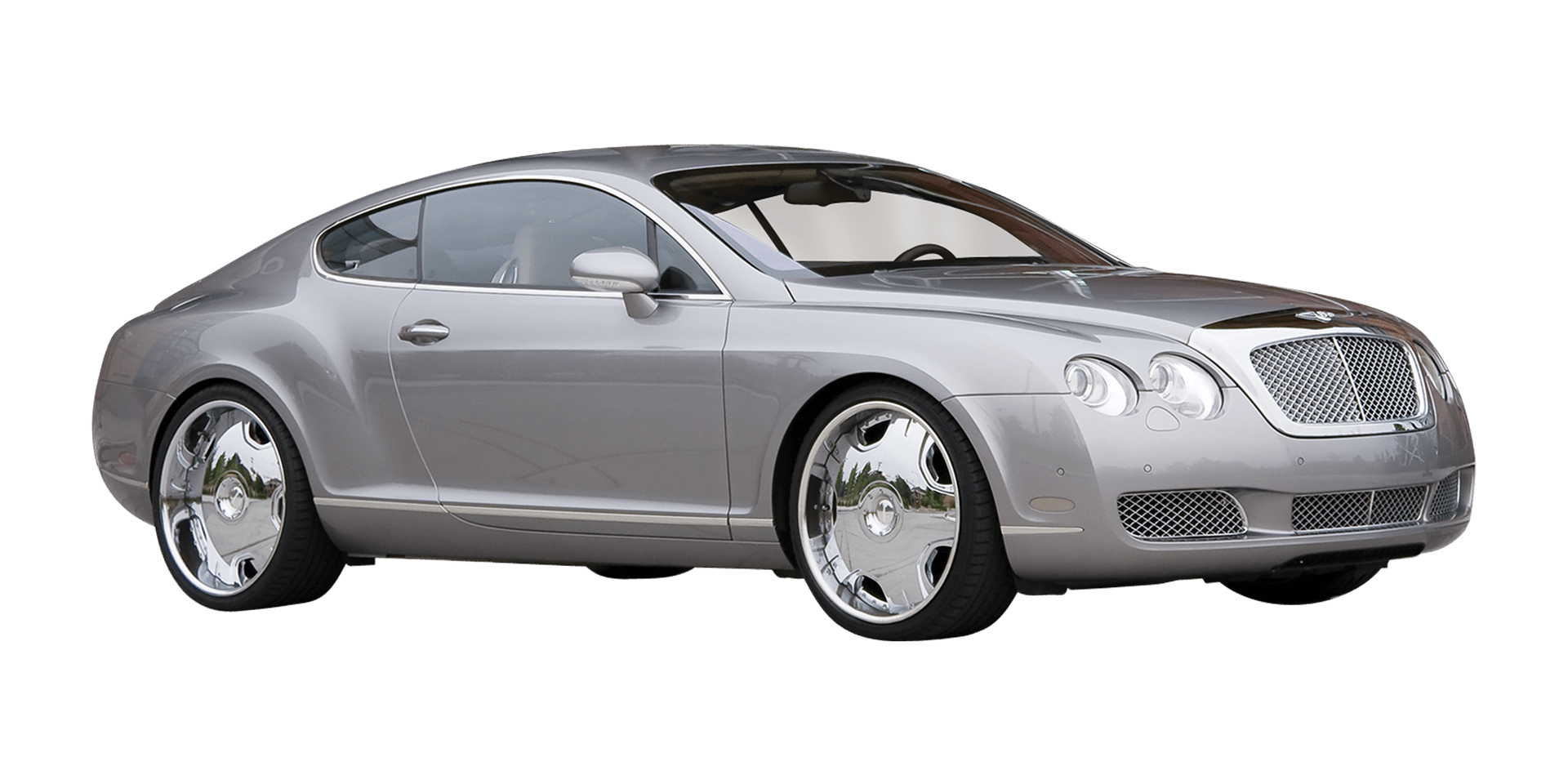More calculations using license plates are coming your way. Here is another fun activity you could try with your child on the road.

## Total It

What You’ll Need

License plates, paper, pencil, and calculator

What to Do

1. As you are travelling in your car or on a bus, each person takes turns calling out a license plate number.
2. All players try to add the numbers in their heads. Talk about what strategies were used in the mental math addition. Were the numbers added by 10’s like 2+8? Were doubles like 6+6 added?
3. Try different problems using the numbers in a license plate. For example, if you use the plate number SJC6321A, ask “Using the numbers on the plate, can you make 5?”
• Can you make 5 using two numbers? “Yes, 3+2 = 5”
• Can you make 5 using three numbers? “Yes, (3+2) x 1 = 5”
• Can you make 5 using four numbers? “Yes, (6+3+1) ÷ 2 = 5”

Try using a calculator to play these games. See if you can solve these problems faster using the calculator.

Parent Pointer: The problem-solving and computational skills your child uses in this activity are very important to mental maths skills, and they also help your child to be creative with numbers.

Reference: http://math.com/